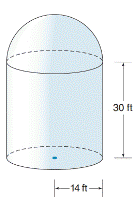Chapter 9.4, Problem 36EElementary Geometry For College St...

7th Edition
Alexander + 2 others
ISBN: 9781337614085

Solutions

Chapter
SectionElementary Geometry For College St...

7th Edition
Alexander + 2 others
ISBN: 9781337614085
Textbook Problem

An observatory has the shape of a right circular cylinder surmounted by a hemisphere. If the radius of the cylinder is 14 ft and its altitude measures 30 ft, what is the surface area of the observatory? If 1 gallon of paint covers 300   f t   2 , how many gallons are needed to paint the surface if it requires two coats? Use your calculator.To determine

To find:

The surface area and number of gallons of paint required to paint an observatory.

Explanation

Formula Used:

The surface area of a hemisphere S = 2 πr2, where r is the radius of the sphere.

Curved or lateral surface area of a cylinder = 2πrh

Volume of cylinder = πr2h

Calculation:

Consider an observatory that has the shape of a right circular cylinder surmounted by a hemisphere.

The base radius of the cylinder (r) =14 ft and, altitude (h) =30 ft.

Surface area of observatory,

= Lateral surface area of right circular cylinder + Surface area of hemisphere

Surface area of observatory=2πrh+2πr2

Surface area of observatory S=2·3

Still sussing out bartleby?

Check out a sample textbook solution.

See a sample solution

The Solution to Your Study Problems

Bartleby provides explanations to thousands of textbook problems written by our experts, many with advanced degrees!

Get Started

Use two disjoint sets to show that 0+2=0.

Mathematical Excursions (MindTap Course List)

28. Find the number A that satisfies for the standard normal z-score.

Mathematical Applications for the Management, Life, and Social Sciences

Evaluate the integral, if it exists. x+2x2+4xdx

Single Variable Calculus: Early Transcendentals, Volume I

For , which vector, a, b, c, or d, best represents F(1, 1)? a b c d

Study Guide for Stewart's Multivariable Calculus, 8th

The solution to y = y2 with y(1)=13 is: a) y=1x+2 b) y=lnx+13 c) y=12x216 d) y=12x16

Study Guide for Stewart's Single Variable Calculus: Early Transcendentals, 8th

Identify possible sources and use them to identify a topic area for research.

Research Methods for the Behavioral Sciences (MindTap Course List)